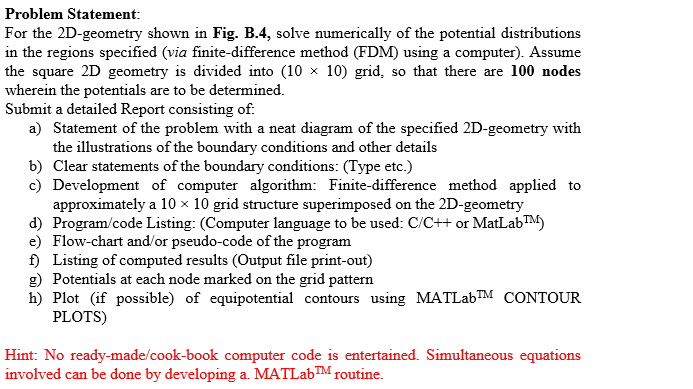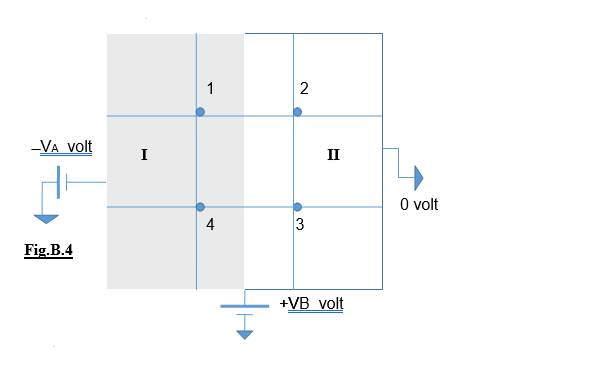# please help i need this as soon as possible this is an electromagnetics fields and w 5153216

This Is An Electromagnetics Fields and Waves Question

Question is Solved Manually Here: (https://www.chegg.com/homework-help/questions-and-answers/problem-b4-shown-fig-b4-square-dielectric-2d-surface-3-cm-x-3-cm-boundary-conditions-indic-q31965506)

We need To Make A Computer Program That Will Do These Calculations As Stated Below.

**************Provide copyable code in C, C++, or Matlab**************Problem Statement For the 2D-geometry shown in Fig. B.4, solve numerically of the potential distributions in the regions specified (via finite-difference method (FDM) using a computer). Assume the square 2D geometry is divided into (10 × 10) grid, so that there are 100 nodes wherein the potentials are to be determined Submit a detailed Report consisting of a) Statement of the problem with a neat diagram of the specified 2D-geometry with the illustrations of the boundary conditions and other details b) Clear statements of the boundary conditions: (Type etc.) c) Development of computer algorithm: Finite-difference method applied to approximately a 10 x 10 grid structure superimposed on the 2D-geometry d) Program/code Listing: (Computer language to be used: C/C++ or MatLabTM) e) Flow-chart and&#39;or pseudo-code of the program f) Listing of computed results (Output file print-out) g) Potentials at each node marked on the grid pattern h) Plot (if possible) of equipotential contours using MATLabTM CONTOUR PLOTS) Hint: No ready-made/cook-book computer code is entertained. Simultaneous equations involved can be done by developing a. MATLabroutine